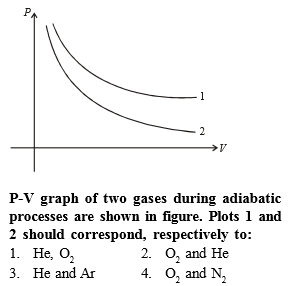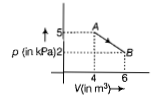You’ve reached the end of your free Videos limit.
#43 | Solved Example: 19
(Physics) > Thermodynamics
Related Practice Questions :Complete Question Bank + Test Series
Complete Question Bank

Difficulty Level:

One mole of an ideal diatomic gas undergoes a transition from A to B along a path AB as shown in the figure.The change in internal energy of the gas during the transition is

(a) 20 kJ

(b) -20 kJ

(c) 20 J

(d) -12 kJ

Complete Question Bank + Test Series
Complete Question Bank

Difficulty Level:

The specific heat of a gas in an isothermal process is -

(1) Infinite

(2) Zero

(3) Negative

(4) Remains constant

Complete Question Bank + Test Series
Complete Question Bank

Difficulty Level:

When an ideal diatomic gas is heated at constant pressure, the fraction of the heat energy supplied which increases the internal energy of the gas, is -

(1) $\frac{2}{5}$

(2) $\frac{3}{5}$

(3) $\frac{3}{7}$

(4) $\frac{5}{7}$

Complete Question Bank + Test Series
Complete Question Bank

Difficulty Level:

A mono atomic gas is supplied the heat Q very slowly keeping the pressure constant. The work done by the gas will be

(1) $\frac{2}{3}Q$

(2) $\frac{3}{5}Q$

(3) $\frac{2}{5}Q$

(4) $\frac{1}{5}Q$